﻿ 基于超混沌映射的位平面彩色图像加密算法 A Novel Hyper-Chaos-Based Colorimage Encryption Algorithm Using Bit-Level Permutation and Diffusion

Computer Science and Application
Vol. 08  No. 09 ( 2018 ), Article ID: 26810 , 14 pages
10.12677/CSA.2018.89150

A Novel Hyper-Chaos-Based Colorimage Encryption Algorithm Using Bit-Level Permutation and Diffusion

Yajuan Li, Ruisong Ye*

Department of Mathematics, Shantou University, Shantou Guangdong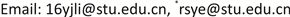Received: Aug. 25th, 2018; accepted: Sep. 7th, 2018; published: Sep. 14th, 2018ABSTRACT

In this paper, a 4D Lorenz map is proposed using in cryptography. Performance evaluations show that it has hyper-chaotic behavior, wide chaotic range and large complexity. Based on this map, a novel image encryption algorithm is designed by employing bit-level permutation and diffusion. In traditional permutation-diffusion structure, the permutation and substitution generally are two independent parts. In this article, encryption algorithm is designed by employing bit-level permutation and diffusion simultaneously. The bit-level permutation is performed by circular shifting, and the bit-level diffusion is carried out by exclusive or (xor) and reverse operations. In addition, to achieve the better ability of resisting chosen-plaintext or known-plaintext attack, the substitution key stream generated using SHA-256 in our method is dependent on the plain image. Consequently, different plain images produce the distinct key stream for substitution. The simulation results and performance analysis show that the proposed image encryption algorithm is both secure and reliable for image encryption.

Keywords:4-D Hyper-Chaotic Maps, Bit-Level, Key Stream Generation1. 引言

1989年，Matthews首次提出基于混沌系统的加密方案  。1997年，Fridrich将混沌映射应用到图像加密系统  。1998年，Fridrich利用2D混沌系统提出置乱–扩散结构的图像加密方案  ，在这种结构下，首先在置换过程中对像素位置进行扰乱，以减少相邻像素之间的强相关性。之后，在扩散过程中像素值逐一改变，后一个值与前一个值相关，前后扩散两轮，类似雪崩效应。现有的图像加密算法中，此结构占据很大部分     。文献  ，首次提出将图像滤波应用在图像加密算法中，首先基于图像块的置乱，扰乱图像像素的相关性，然后在扩散过程中，使用它提出的图像滤波器的方法，对置乱后的图像进行扩散，最后部分的仿真测试显示它比一般的图像加密方案要更优良。文献  ，采用的是2D henon映射加密图像，打破了传统的置乱–扩散结构，它采用两点式置乱扩散同时进行，大大的提升了密码系统的速度，并且为了抵抗选择明文和已知明文攻击，它采用了根据明文生成密钥流的方式，即不同的原图将会产生完全不同的密钥流。文献   ，均采用2D映射，生成混沌序列，应用在加密图像中，  基于级联调制耦合(CMC)模型提出一种新的2D Logistic ICMIC耦合映射(2D-LICM)，比起参数较少，轨道相对而言较简单的一维混沌映射，它的效果会更加优良，  介绍的是一种新的2维Sine Logistic模型映射(2D-SLMM)，它起源于Sine映射和Logistic映射，比起单独的两个映射，2D-SLMM具有较宽的混沌范围、较好的遍历性和超混沌特性。与1D混沌映射相比，它们通常包含一个变量和几个参数，并且它们的轨道很简单，因此它们的参数和初始值相对而言容易地估计,当这些映射被应用在图像加密系统中，容易被破解  。另一方面，高维混沌映射具有更多的变量和参数，通常表现出良好的超混沌性质，更适合于加密。

2. Hyper-Lorenz混沌系统

$\begin{array}{l}\stackrel{˙}{x}=a\left(y-x\right)+w\\ \stackrel{˙}{y}=cx-y-xz\\ \stackrel{˙}{z}=xy-bz\\ \text{w}=-yz+rw\end{array}$ (1)

${w}_{0}\in \left(-250,250\right)$ ，这些初始值将被用来当作整个系统的密钥。当 $a=10,b=\frac{8}{3},c=28,-1.52\le r\le -0.06$ 时，

3. 系统结构

3.1. 密钥产生器

${d}_{1}=\frac{hex2dec\left({h}_{i}\right)}{{2}^{64}},i=1,\cdots ,4$ . (2)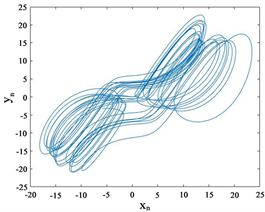(a)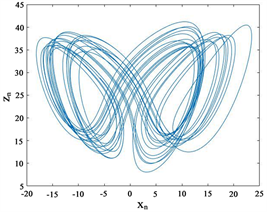(b)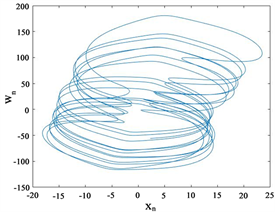(c)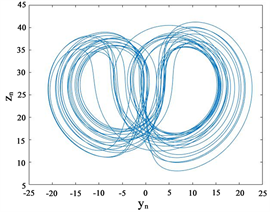(d)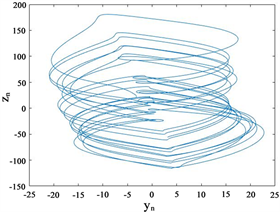(e)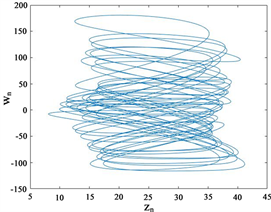(f)

Figure 1. Projections of the chaotic attractor of system (1), (a) x – y; (b) x – z; (c) x – w; (d) y – z; (e) y – w; (f) z – wFigure 2. The Lyapunov exponent diagram of the (1)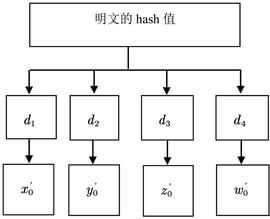Figure 3. The method to generate keys

$s={x}_{0}+{y}_{0}+{z}_{0}+{w}_{0}\mathrm{mod}1$ . (3)

$\begin{array}{l}{x}^{0}={d}_{1}+{x}_{0}+s\mathrm{mod}40;\\ {y}^{0}={d}_{2}+{y}_{0}+s\mathrm{mod}40;\\ {z}^{0}={d}_{3}+{z}_{0}+s\mathrm{mod}81;\\ {w}^{0}={d}_{4}+{w}_{0}+s\mathrm{mod}250\end{array}$ (4)

3.2. 加密算法

1. 设置超混沌Lorenz映射的参数a = 10, b = 8/3, c = 28, r = −1；

2. 根据3.1，跟新初始值x0，y0，z0，w0

3. 用更新后的初始值x0，y0，z0，w0，对超混沌Lorenz映射迭代，并得到4个序列x1，y1，z1，w1，再更新4个序列：x1 = x1 − ⌊x1⌋，y1=y1 − ⌊y1⌋，z1=z1 − ⌊z1⌋，w1=w1 − ⌊w1⌋，其中⌊ ⌋表示四舍五入。

4. 选取x，y，z，w：x = x1(L + 1:L + 1 + M × N)，y = y1(L + 1:L + 1 + M × N)，z = z1(L + 1:L + 1 + M × N)，w = w1(L + 1:L + 1 + M × N)；其中M，N表示明文大小。

1. 得到明文的三个通道R，G，B，并由BBD分解得到比特平面图像：Rbit，Gbit，Bbit；

2. 将Rbit，Gbit，Bbit分别变成rM,8N，gM,8N，bM,8N，并初始化矩阵RBM,8N，GBM,8N，BBM,8N

3. 处理混沌序列x，y，z，令X = x，X = floor(X × 1014) mod 256，再将X用比特平面表示，最后将X转换为大小为M × 8N，y，z也做同样操作，得到X，Y，Z；

4. for i=1:M

5. kr = floor(abs(x(i))×105 ) mod M 对R通道的置乱扩散，floor表示向下取整；

6. tr = circshift(r(i,:)', kr)' circshift表示循环移位函数；

7. if i==1

8. RB(i,:)=bitxor(tr,r(end,:)) bitxor表示异或；

9. else

10. RB(i,:)=bitxor(bitxor(tr,RB(i-1,:)),X(i,:));

11. end

12. if w(i)>0

13. RB(i,:)=fliplr(RB(i,:) fliplr表示取反；

14. end

15. kg = floor(abs(y(i))×105) mod M对G通道的置乱扩散；

16. tg = circshift(g(i,:)', kg)'

17. if i==1

18. GB(i,:)=bitxor(tg,g(end,:))bitxor表示异或；

19. else

20. GB(i,:)=bitxor(bitxor(tg,GB(i-1,:)),Y(i,:));

21. end

22. if w(i)<0.2

23. GB(i,:)=fliplr(GB(i,:)；

24. end

25. kb = floor(abs(z(i))×105) mod M 对B通道的置乱扩散；

26. tb = circshift(b(i,:)', kb)'

27. if i==1

28. BB(i,:)=bitxor(tb,b(end,:))；

29. else

30. BB(i,:)=bitxor(bitxor(tb,BB(i-1,:) ),Z(i,:));

31. end

32. 接下来对三个通道(R，G，B)的列进行类似的移位异或取反操作，这边仅给出 通道，其余类似不再赘叙；

33. 初试化矩阵RBCM,8N，GBCM,8N，BBCM,8N，令A = 8 × N；

34. for i=1:8×N

35. kr = floor(abs(y(i))×108) mod A；；

36. tr = circshift(RB(:,i), kr)；

37. i==1

38. RBC(:,i)=bitxor(tr,RB(:,end))；

39. else

40. RBC(:,i)=bitxor(bitxor(tr,RBC(:,i-1)),Y(:,i))；

41. end

42. if w(i)>0

43. RBC(:,i)=fliplr(RBC(:,i)；

44. end

45. 再对G，B通道做类似的操作；

46. end

47.分别将RBC，GBC，BBC转换为像素平面矩阵，再组合成一幅密图C。

4. 仿真实验和性能分析Figure 4. The encryption process of R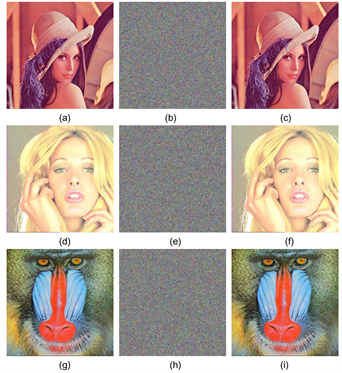Figure 5. (a) - (h) correspond to the plain-text, cipher-text image and decrypted image of Lena (a), Tiffany (d), Mandrill (g), respectively

$\text{Key}=\left[{x}_{0}=1.751231;{y}_{0}=1.53262871;{z}_{0}=13.18344121;{w}_{0}=1.627362;L=2000\right]$

4.1. 统计分析

4.1.1. 直方图分析

4.1.2. 像素间的相关性分析

${r}_{xy}=\frac{\mathrm{cov}\left(u,v\right)}{\sqrt{D\left(u\right)}\sqrt{D\left(v\right)}}$ (5)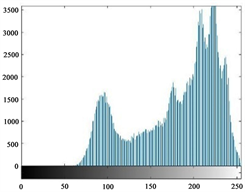(a)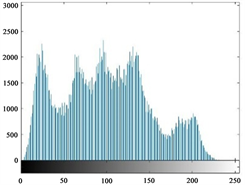(b)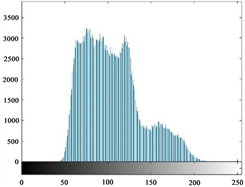(c)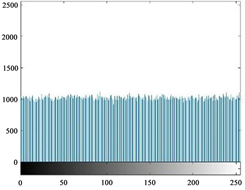(d)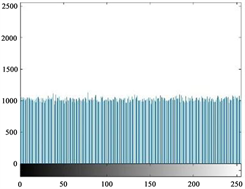(e)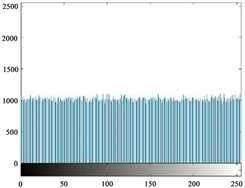(f)

Figure 6. (a) - (f) are the histograms of the red, green, and blue color channels of the Lena plaintext image and its cipher-text, respectively

$\mathrm{cov}\left(u,v\right)=\frac{1}{N}{\sum }_{i=1}^{N}\left({x}_{i}-E\left(u\right)\right)\left({y}_{i}-E\left(v\right)\right)$ (6)

$D\left(u\right)=\frac{1}{N}{\sum }_{i=1}^{N}{\left({u}_{i}-E\left(u\right)\right)}^{2}$ (7)

$E\left(u\right)=\frac{1}{N}{\sum }_{i=1}^{N}{u}_{i}$ (8)

4.1.3. 图像信息熵分析Table 1. The correlation coefficient between Plain image and its cipher-text in horizontal, vertical and diagonal directions, respectively.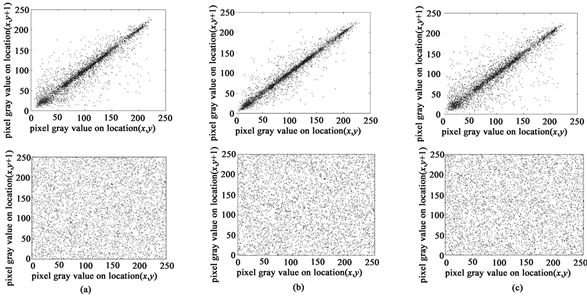Figure 7. (a) - (c) are the distributions of plain-text and cipher-text pixels in horizontal, vertical and diagonal directions, respectively

$H\left(m\right)={\sum }_{i=0}^{L-1}p\left({m}_{i}\right){\mathrm{log}}_{2}^{p\left({m}_{i}\right)},\text{\hspace{0.17em}}\text{\hspace{0.17em}}{\sum }_{i=0}^{L-1}p\left({m}_{i}\right)=1$ (9)

4.2. 密钥空间

4.3. 密钥敏感性

$\text{key}=\left[{x}_{0}=1.751231;{y}_{0}=1.53262871;{z}_{0}=13.18344121;{w}_{0}=1.627362;L=2000\right]$

$\text{key1}=\left[{x}_{0}=1.75123100000001;{y}_{0}=1.53262871;{z}_{0}=13.18344121;{w}_{0}=1.627362;L=2000\right]$

$\text{key2}=\left[{x}_{0}=1.751231;{y}_{0}=1.53262871000001;{z}_{0}=13.18344121;{w}_{0}=1.627362;L=2000\right]$

$\text{key3}=\left[{x}_{0}=1.751231;{y}_{0}=1.53262871;{z}_{0}=13.18344121000001;{w}_{0}=1.627362;L=2000\right]$

$\text{key4}=\left[{x}_{0}=1.751231;{y}_{0}=1.53262871;{z}_{0}=13.18344121;{w}_{0}=1.62736200000001;L=2000\right]$Table 3. The information entropy of each color channel of different plain-texts and cipher-text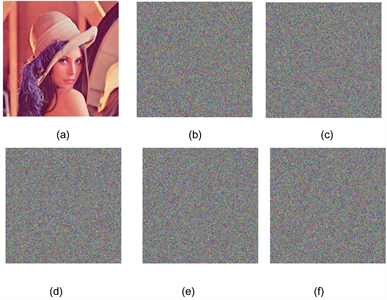Figure 8. Encryption sensitivity test. (a) - (f) were decrypted with key, key1 - key 5, respectivelyTable 4. The NPCR and UACI for decrypted image using different keys (Lena) (%)

$\text{key5}=\left[{x}_{0}=1.751231;{y}_{0}=1.53262871;{z}_{0}=13.18344121;{w}_{0}=1.627362;L=2001\right]$

4.4. 差分分析

$\text{NPCR}=\frac{1}{W×H}{\sum }_{i=0}^{H}{\sum }_{j=0}^{W}D\left(i,j\right)×100%$ (10)

$\text{UACI}=\frac{1}{W×H}{\sum }_{i=0}^{H}{\sum }_{j=0}^{W}\frac{|{c}_{1}\left(i,j\right)-{c}_{2}\left(i,j\right)|}{255}$ (11)

$D\left(i,j\right)=\left\{\begin{array}{l}0\text{\hspace{0.17em}}\text{\hspace{0.17em}}\text{if}\text{\hspace{0.17em}}{c}_{1}\left(i,j\right)={c}_{2}\left(i,j\right)\\ 1\text{\hspace{0.17em}}\text{\hspace{0.17em}}\text{ }\text{if}\text{\hspace{0.17em}}{c}_{1}\left(i,j\right)\ne {c}_{2}\left(i,j\right)\end{array}$ (12)

$W,H$ 分别是图像的行数和列数， ${c}_{1}\left(i,j\right),{c}_{2}\left(i,j\right)$ 分别是对应需要被衡量的两幅图像，本文指用同一个密钥加密仅改变一个明文像素值的两幅密图。理论上来说两幅随机图像的NPCR，UACI理论期望值分别约为99.6094%，33.4635%，本文将用彩色图像Lena和Mandrill来完成差分实验，对于每一个实验图片，将随机选取1000个像素点，每次改变一个像素值，得到一个新的图像，然后用同样的密钥去加密两个相差仅一个像素的原密钥加密仅改变一个明文像素值的两幅密图。理论上来说两幅随机图像的NPCR，UACI理论期望值分别约为99.6094%，33.4635%，本文将用彩色图像Lena和Mandrill来完成差分实验，对于每一个实验图片，将随机选取1000个像素点，每次改变一个像素值，得到一个新的图像，然后用同样的密钥去加密两个相差仅一个像素的原图，实验数据结果呈现在表5表6以及NPCR，UACI的曲线变化图如图9所示。期望值分别约为99.6094%，33.4635%，本文将用彩色图像Lena和Mandrill来完成差分实验，同时对于明文Lena图像的每个颜色通道，随机地选取的100个像素值，对每个灰度值仅随机改变1个像素值，然后用本文提出加密算法去加密改变前后的明文图像Lena，得到对应每个颜色通道的100个NPCR，UACI曲线变化图如图10所示，实验数据结果呈现在表7。实验结果表明，本文提出的算Table 5. The NPCR results for different image (%)Table 6. The UACI results for different image (%)Table 7. The maximum, minimum, and average values of the NPCR and UACI sequences between the corresponding cipher-texts before and after the Lena image changes (%)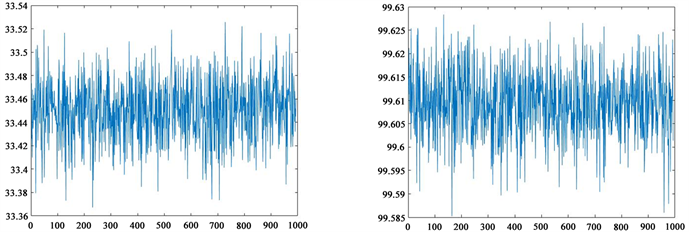(a) (b)

Figure 9. The NPCR and UACI of 1000 images that only change one pixel. (a) NPCR, (b) UACI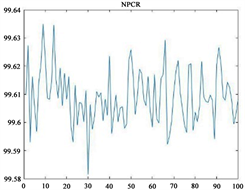(a)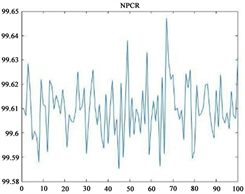(b)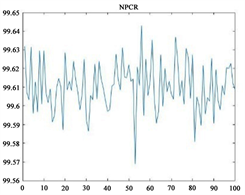(c)(d)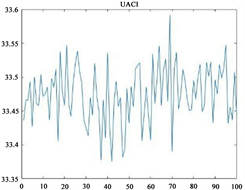(e)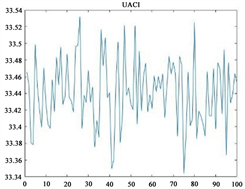(f)

Figure 10. (a) - (c) and (d) - (f) are the NPCR and UACI curves of the cipher-text obtained before and after the change of the plaintext image, respectively

5. 总结

A Novel Hyper-Chaos-Based Colorimage Encryption Algorithm Using Bit-Level Permutation and Diffusion[J]. 计算机科学与应用, 2018, 08(09): 1382-1395. https://doi.org/10.12677/CSA.2018.89150

1. 1. 21世纪经济报道. 新加坡史上最严重个人数据泄露事件——李显龙称是“冲他来的”[EB/OL]. https://m.21jingji.com/article/20180723/herald/e830a398a17071d0c91657d3088969d1.html, 2018-7-26.

2. 2. Li, S., Chen, G., Cheung, A., Bhargava, B. and Lo, K.T. (2007) On the Design of Perceptual MPEG-Video Encryption Algorithms. IEEE Transactions on Circuits and Systems for Video Technology, 17, 214-223. https://doi.org/10.1109/TCSVT.2006.888840

3. 3. Li, Y., Wang, C. and Chen, H. (2017) A Hyper-Chaos-Based Image Encryp-tion Algorithm Using Pixel-Level Permutation and Bit-Level Permutation. Optics and Lasers in Engineering, 90, 238-246. https://doi.org/10.1016/j.optlaseng.2016.10.020

4. 4. Chen, J., Zhu, Z.L., Zhang, L.B., Zhang, Y. and Yang, B.Q. (2018) Ex-ploiting Self-Adaptive Permutation-Diffusion and DNA Random Encoding for Secure and Efficient Image Encryption. Signal Pro-cessing, 142, 340-353. https://doi.org/10.1016/j.sigpro.2017.07.034

5. 5. Zhang, Y.Q., Wang, X.Y., Liu, J. and Chi, Z.L. (2016) An Image Encryption Scheme Based on the MLNCML System Using DNA Sequences. Optics and Lasers in Engineering, 82, 95-103. https://doi.org/10.1016/j.optlaseng.2016.02.002

6. 6. Zhang, X. and Wang, X. (2017) Multiple-Image Encryption Algorithm Based on Mixed Image Element and Chaos. Computers & Electrical Engineering, 62, 401-413. https://doi.org/10.1016/j.compeleceng.2016.12.025

7. 7. Zhu, W., Yang, G., Chen, L. and Xu, J. (2014) Multiple-Image Encryp-tion Based on Wavelet Transform and Improved Double Random Phase Encoding. Nanjing University of Posts & Telecommunications, 34, 87-92.

8. 8. Hua, Z. and Zhou, Y. (2017) Design of Image Cipher Using Block-Based Scrambling and Image Filtering. Infor-mation Sciences, 396, 97-113. https://doi.org/10.1016/j.ins.2017.02.036

9. 9. Zhu, H., Zhao, C., Zhang, X. and Yang, L. (2014) An Image Encryption Scheme Using Generalized Arnold Map and Affine cipher. Optik-International Journal for Light and Electron Optics, 125, 6672-6677. https://doi.org/10.1016/j.ijleo.2014.06.149

10. 10. Zhu, Z.-L., Zhang, W., Wong, K.-W. and Yu, H. (2011) A Chaos-Based Sym-metric Image Encryption Scheme Using a Bit-Level Permutation. Information Sciences, 181, 1171-1186. https://doi.org/10.1016/j.ins.2010.11.009

11. 11. Matthews, R. (1989) On the Derivation of a “Chaotic” Encryption Algorithm. Cryptologia, 13, 29-42. https://doi.org/10.1080/0161-118991863745

12. 12. Fridrich, J. (1997) Image Encryption Based on Chaotic Maps. In Systems, Man, and Cybernetics, 1997. IEEE International Conference on Computational Cybernetics and Simulation, 2, 1105-1110. https://doi.org/10.1109/ICSMC.1997.638097

13. 13. Fridrich, J. (1998) Symmetric Ciphers Based on Two-Dimensional Chaotic Maps. International Journal of Bifurcation and Chaos, 8, 1259-1284. https://doi.org/10.1142/S021812749800098X

14. 14. Ping, P., Xu, F., Mao, Y. and Wang, Z. (2018) Designing Permutation-Substitution Image Encryption Networks with Henon Map. Neurocom-puting, 283, 53-63. https://doi.org/10.1016/j.neucom.2017.12.048

15. 15. Cao, C., Sun, K. and Liu, W. (2018) A Novel Bit-Level Image Encryption Algorithm Based on 2D-LICM Hyperchaotic Map. Signal Processing, 143, 122-133. https://doi.org/10.1016/j.sigpro.2017.08.020

16. 16. Hua, Z., Zhou, Y., Pun, C.M. and Chen, C.P. (2015) 2D Sine Logistic Modula-tion Map for Image Encryption. Information Sciences, 297, 80-94.

17. 17. Wang, H., Xiao, D., Chen, X. and Huang, H. (2018) Crypta-nalysis and Enhancements of Image Encryption Using Combination of the 1D Chaotic Map. Signal Processing, 144, 444-452.

18. 18. Liao, X., Hahsmi, M.A. and Haider, R. (2018) An Efficient Mixed Inter-Intra Pixels Substitution at 2bits-Level for Im-age Encryption Technique Using DNA and Chaos. International Journal for Light and Electron Optics, 153, 117-134. https://doi.org/10.1016/j.ijleo.2017.09.099

19. 19. Wang, X.Y., Zhang, Y.Q. and Bao, X.M. (2015) A Novel Chaotic Image En-cryption Scheme Using DNA Sequence Operations. Optics and Lasers in Engineering, 73, 53-61. https://doi.org/10.1016/j.optlaseng.2015.03.022

20. 20. Li, X., Wang, L., Yan, Y. and Liu, P. (2016) An Improvement Color Image Encryption Algorithm Based on DNA Operations and Real and Complex Chaotic Systems. International Journal for Light and Elec-tron Optics, 127, 2558-2565. https://doi.org/10.1016/j.ijleo.2015.11.221

21. 21. Liao, X., Kulsoom, A. and Ullah, S. (2016) A Modified (Dual) Fusion Technique for Image Encryption Using SHA-256 Hash and Multiple Chaotic Maps. Multimedia Tools and Applications, 75, 11241-11266. https://doi.org/10.1007/s11042-015-2851-7

22. 22. Liu, H. and Wang, X. (2012) Image Encryption Using DNA Complementary Rule and Chaotic Maps. Applied Soft Computing, 12, 1457-1466. https://doi.org/10.1016/j.asoc.2012.01.016

23. 23. Zhou, Y., Cao, W. and Chen, C.P. (2014) Image Encryption Using Binary Bitplane. Signal Processing, 100, 197-207. https://doi.org/10.1016/j.sigpro.2014.01.020

24. 24. Pak, C. and Huang, L. (2017) A New Color Image Encryption Using Combina-tion of the 1D Chaotic Map. Signal Processing, 138, 129-137. https://doi.org/10.1016/j.sigpro.2017.03.011

25. NOTES

*通讯作者。